# CIE A Level Maths: Pure 3复习笔记8.3.1 Exponential Form of Complex Numbers

### Exponential Form of Complex Numbers

You now know how to do lots of operations with complex numbers: add, subtract, multiply, divide, raise to a power and even square root. The last operation to learn is raising the number e to the power of an imaginary number.

#### How do we calculate e to the power of an imaginary number?

• Given an imaginary number (iθ) we can define exponentiation as
•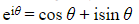•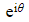is the complex number with modulus 1 and argument θ
• This works with our current rules of exponents
•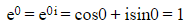• This shows e to the power 0 would still give the answer of 1
•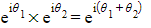• This is because when you multiply complex numbers you can add the arguments
• This shows that when you multiply two powers you can still add the indices
•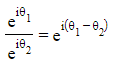• This is because when you divide complex numbers you can subtract the arguments
• This shows that when you divide two powers you can still subtract the indices

#### What is the exponential form of a complex number?

• Any complex number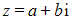can be written in polar form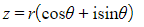• r is the modulus
• θ is the argument
• Using the definition of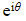we can now also write Z in exponential form
•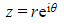#### Why do I need to use the exponential form of a complex number?

• It's just a shorter and quicker way of expressing complex numbers
• It makes a link between the exponential function and trigonometric functions
• It makes it easier to remember what happens with the moduli and arguments when multiplying and dividing
• If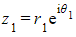and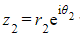then
•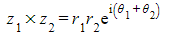• You can clearly see that the moduli have been multiplied and the arguments have been added
•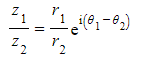• You can clearly see that the moduli have been divided and the arguments have been subtracted

#### What are some common numbers in exponential form?

• Asand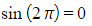you can write:
•• Using the same idea you can write:
•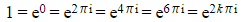where k is any integer
• As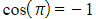and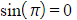you can write:
•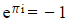• Or more commonly written as
•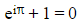• Asand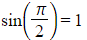you can write:
•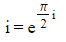#### Worked Example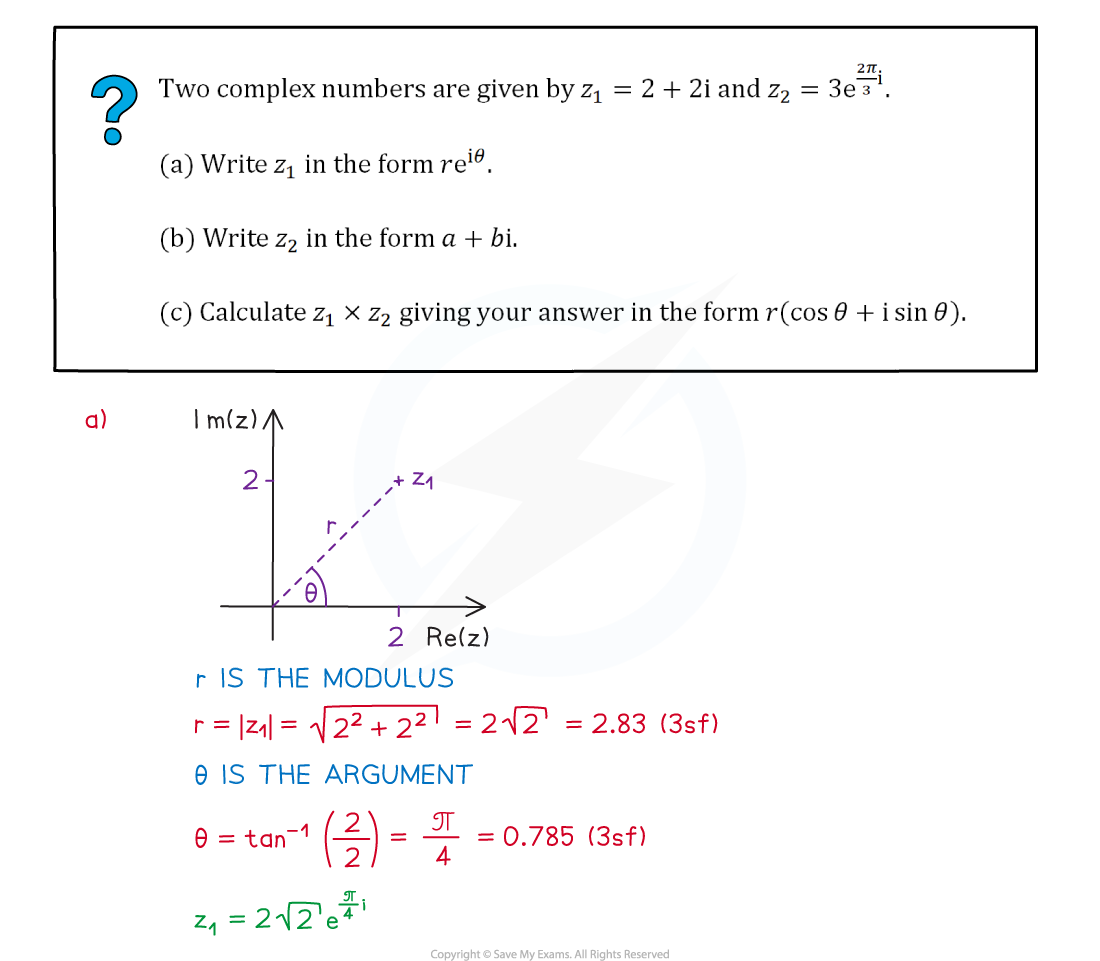#### Exam Tip

• The powers can be long and contain fractions so make sure you write the expression clearly.
• You don’t want to lose marks because the examiner can’t read your answer